# How to count cells with text in Excel: any, specific, filtered cells

How do I count cells with text in Excel? There are a few different formulas to count cells that contain any text, specific characters or only filtered cells. All the formulas work in Excel 2019, 2016, 2013 and 2010.

Initially, Excel spreadsheets were designed to work with numbers. But these days we often use them to store and manipulate text too. Want to know how many cells with text there are in your worksheet? Microsoft Excel has several functions for this. Which one should you use? Well, it depends on the situation. In this tutorial, you will find a variety of formulas and when each formula is best to be used.

## How to count number of cells with text in Excel

There are two basic formulas to find how many cells in a given range contain any text string or character.

### COUNTIF formula to count all cells with text

When you wish to find the number of cells with text in Excel, the COUNTIF function with an asterisk in the criteria argument is the best and easiest solution:

COUNTIF(range, "*")

Because the asterisk (*) is a wildcard that matches any sequence of characters, the formula counts all cells that contain any text.

### SUMPRODUCT formula to count cells with any text

Another way to get the number of cells containing text is to combine the SUMPRODUCT and ISTEXT functions:

SUMPRODUCT(--ISTEXT(range))

Or

SUMPRODUCT(ISTEXT(range)*1)

The ISTEXT function checks if each cell in the specified range contains any text characters and returns an array of TRUE (cells with text) and FALSE (other cells) values. The double unary (--) or the multiplication operation coerces TRUE and FALSE into 1 and 0, respectively, producing an array of ones and zeros. The SUMPRODUCT function sums all the elements of the array and returns the number of 1's, which is the number of cells that contain text.

To gain more understanding of how these formulas work, please see which values are counted and which are not:

 What is counted What is not counted Cells with any text Special characters Numbers formatted as text Visually blank cells that contain an empty string (""), apostrophe ('), space or non-printing characters Numbers Dates Logical values of TRUE and FALSE Errors Blank cells

For example, to count cells with text in the range A2:A10, excluding numbers, dates, logical values, errors and blank cells, use one of these formulas:

`=COUNTIF(A2:A10, "*")`

`=SUMPRODUCT(--ISTEXT(A2:A10))`

`=SUMPRODUCT(ISTEXT(A2:A10)*1)`

The screenshot below shows the result: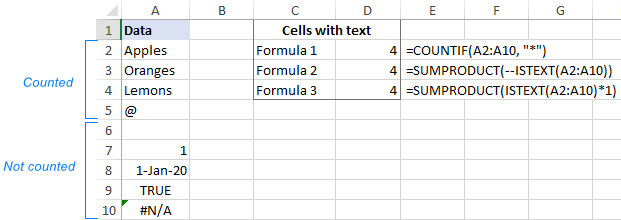## Count cells with text excluding spaces and empty strings

The formulas discussed above count all cells that have any text characters in them. In some situations, however, that might be confusing because certain cells may only look empty but, in fact, contain characters invisible to the human eye such as empty strings, apostrophes, spaces, line breaks, etc. As a result, a visually blank cell gets counted by the formula causing a user to pull out their hair trying to figure out why :)

To exclude "false positive" blank cells from the count, use the COUNTIFS function with the "excluded" character in the second criterion.

For example, to count cells with text in the range A2:A7 ignoring those that contain a space character, use this formula:

`=COUNTIFS(A2:A7,"*", A2:A7, "<> ")`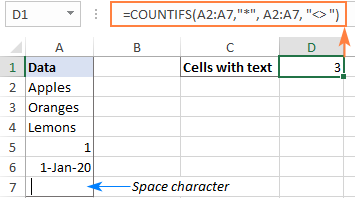If your target range contains any formula-driven data, some of the formulas may result in an empty string (""). To ignore cells with empty strings too, replace "*" with "*?*" in the criteria1 argument:

`=COUNTIFS(A2:A9,"*?*", A2:A9, "<> ")`

A question mark surrounded by asterisks indicates that there should be at least one text character in the cell. Since an empty string has no characters in it, it does not meet the criteria and is not counted. Blank cells that begin with an apostrophe (') are not counted either.

In the screenshot below, there is a space in A7, an apostrophe in A8 and an empty string (="") in A9. Our formula leaves out all those cells and returns a text-cells count of 3: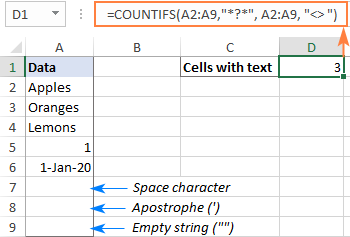## How to count cells with certain text in Excel

To get the number of cells that contain certain text or character, you simply supply that text in the criteria argument of the COUNTIF function. The below examples explain the nuances.

To match the sample text exactly, enter the full text enclosed in quotation marks:

COUNTIF(range, "text")

To count cells with partial match, place the text between two asterisks, which represent any number of characters before and after the text:

COUNTIF(range, "*text*")

For example, to find how many cells in the range A2:A7 contain exactly the word "bananas", use this formula:

`=COUNTIF(A2:A7, "bananas")`

To count all cells that contain "bananas" as part of their contents in any position, use this one:

`=COUNTIF(A2:A7, "*bananas*")`

To make the formula more user-friendly, you can place the criteria in a predefined cell, say D2, and put the cell reference in the second argument:

`=COUNTIF(A2:A7, D2)`

Depending on the input in D2, the formula can match the sample text fully or partially:

• For full match, type the whole word or phrase as it appears in the source table, e.g. Bananas.
• For partial match, type the sample text surrounded by the wildcard characters, like *Bananas*.

As the formula is case-insensitive, you may not bother about the letter case, meaning that *bananas* will do as well.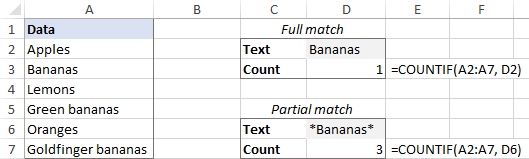Alternatively, to count cells with partial match, concatenate the cell reference and wildcard characters like:

`=COUNTIF(A2:A7, "*"&D2&"*")`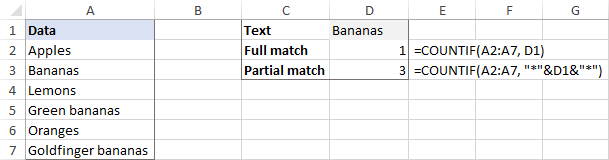## How to count filtered cells with text in Excel

When using Excel filter to display only the data relevant at a given moment, you may sometimes need to count visible cells with text. Regrettably, there is no one-click solution for this task, but the below example will comfortably walk you through the steps.

Supposing, you have a table like shown in the image below. Some entries were pulled from a larger database using formulas, and various errors occurred along the way. You are looking to find the total number of items in column A. With all the rows visible, the COUNTIF formula that we've used for counting cells with text works a treat:

`=COUNTIF(A2:A10, "*")`

And now, you narrow down the list by some criteria, say filter out the items with quantity greater than 10. The question is – how many items remained?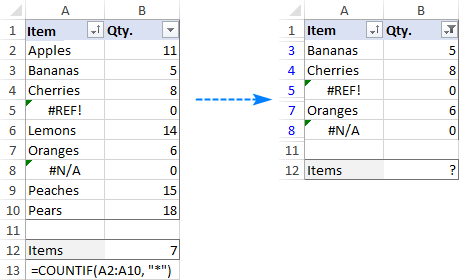To count filtered cells with text, this is what you need to do:

1. In your source table, make all the rows visible. For this, clear all filters and unhide hidden rows.
2. Add a helper column with the SUBTOTAL formula that indicates if a row is filtered or not.
To handle filtered cells, use 3 for the function_num argument:

`=SUBTOTAL(3, A2)`

To identify all hidden cells, filtered out and hidden manually, put 103 in function_num:

`=SUBTOTAL(103, A2)`

In this example, we want to count only visible cells with text regardless of how other cells were hidden, so we enter the second formula in A2 and copy it down to A10.

For visible cells, the formula returns 1. As soon as you filter out or manually hide some rows, the formula will return 0 for them. (You won't see those zeros because they are returned for hidden rows. To make sure it works this way, just copy the contents of a hidden cell with the Subtotal formula to any visible say, say =D2, assuming row 2 is hidden.)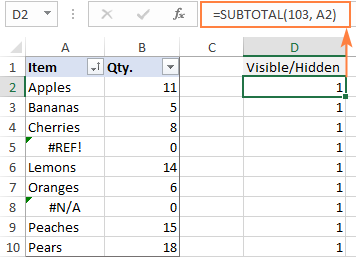3. Use the COUNTIFS function with two different criteria_range/criteria pairs to count visible cells with text:
• Criteria1 - searches for cells with any text ("*") in the range A2:A10.
• Criteria2 - searches for 1 in the range D2:D10 to detect visible cells.

`=COUNTIFS(A2:A10, "*", D2:D10, 1)`

Now, you can filter the data the way you want, and the formula will tell you how many filtered cells in column A contain text (3 in our case):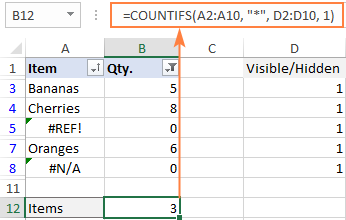If you'd rather not insert an additional column in your worksheet, then you will need a longer formula to accomplish the task. Just choose the one you like better:

`=SUMPRODUCT(SUBTOTAL(103, INDIRECT("A"&ROW(A2:A10))), --(ISTEXT(A2:A10)))`

`=SUMPRODUCT(SUBTOTAL(103, OFFSET(A2:A10, ROW(A2:A10) - MIN(ROW(A2:A10)),,1)), -- (ISTEXT(A2:A10)))`

The multiplication operator will work as well:

`=SUMPRODUCT(SUBTOTAL(103, INDIRECT("A"&ROW(A2:A10))) * (ISTEXT(A2:A10)))`

`=SUMPRODUCT(SUBTOTAL(103, OFFSET(A2:A10, ROW(A2:A10)-MIN(ROW(A2:A10)),,1)) * (ISTEXT(A2:A10)))`

Which formula to use is a matter of your personal preference - the result will be the same in any case: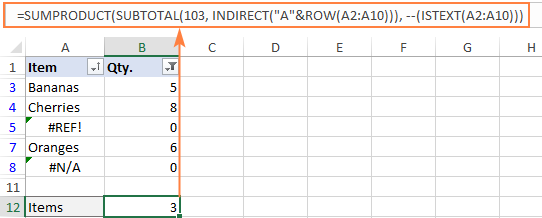### How these formulas work

The first formula employs the INDIRECT function to "feed" the individual references of all cells in the specified range to SUBTOTAL. The second formula uses a combination of the OFFSET, ROW and MIN functions for the same purpose.

The SUBTOTAL function returns an array of 1's and 0's where ones represent visible cells and zeros match hidden cells (like the helper column above).

The ISTEXT function checks each cell in A2:A10 and returns TRUE if a cell contains text, FALSE otherwise. The double unary operator (--) coerces the TRUE and FALSE values into 1's and 0's. At this point, the formula looks as follows:

`=SUMPRODUCT({0;1;1;1;0;1;1;0;0}, {1;1;1;0;1;1;0;1;1})`

The SUMPRODUCT function first multiplies the elements of both arrays in the same positions and then sums the resulting array.

As multiplying by zero gives zero, only the cells represented by 1 in both arrays have 1 in the final array.

`=SUMPRODUCT({0;1;1;0;0;1;0;0;0})`

And the number of 1's in the above array is the number of visible cells that contain text.

That's how to how to count cells with text in Excel. I thank you for reading and hope to see you on our blog next week!

Excel formulas to count cells with text

### 18 responses to "How to count cells with text in Excel: any, specific, filtered cells"

1.Talal says:

Sir
I have row of duty roaster which contains DO RD, and I wanted to count number of DO till just it change to RD then count the number RD till just change to OD then again counting the remaining ODs for the rest of the month.
I can sen you my time sheet for clearer info.

•Alexander Trifuntov (Ablebits.com Team) says:

Hello Talal!
Could you please describe your task in more detail and send us a small sample workbook with the source data and expected result to support@ablebits.com? Please shorten your tables to 10-20 rows/columns and include the link to your blog comment.

2.J. Merry says:

Good Day Sir,
My question is this. I have a list of all "Fruits" in column A. Those "Fruits" count for many row entries each. I would like to count how many rows each "Fruit" totals to. Lets say I have "Apple" 50 times, "Banana" 20 Times and each "Fruit" has a different number of appearances and there are a LOT of different "Fruit". My goal is to create a list of how many times the "Fruit" shows on my spreadsheet in a certain Column and output a count by Type of "Fruit". So I get a list of How many times "Apple" shows or "Banana" Shows.

3.zech says:

hi. Need help.
I have a row of numbers and text "IN" (in different cells).
What formula do I use to count the total if any of the cells in the row have numbers and IN.

•Alexander Trifuntov (Ablebits.com Team) says:

Hello!

4.Seven says:

hello sir,
i have a 03 criterias (higher,lower and standard)in a column which ranges from (8-34200 rows)i also have date column now my problem is whenever i makes a filter in date for example if i want to check how many higher ,lower cases are occured on particular date

•Alexander Trifuntov (Ablebits.com Team) says:

Hello!
I’m sorry but your task is not entirely clear to me. For me to be able to help you better, please describe your task in more detail. Please specify what you were trying to find, what formula you used and what problem or error occurred. Give an example of the source data and the expected result.
It’ll help me understand it better and find a solution for you. Thank you.

•Alexander Trifuntov (Ablebits.com Team) says:

Hello!
If I understand your problem correctly, you need to use the COUNTIFS function to count. Read more here.

5.Hudson says:

Is there a formula that will count all cells containing text within a specific range except cells that contain certain a certain word or character (in my case, I need a count that includes everything in the spreadsheet except blank cells and cells that contain "X"). Thank you!

6.Joetta says:

Im looking for a formula to give me only the number of managers I have working projects. Each manager has multiple projects they work on. Manager names are in column A. Projects in B. I only want to know the total managers I have, not the projects I have. For instance, John's name is listed 8 times, Mike's name is listed 6 and Jill's name is listed 7 times. The answer I want is 3.

7.Matt Sherry says:

Hello, I'm trying to get a row to tally if a range has either P,S, or 2 within the range. Is there a way to accomplish this?

•Alexander Trifuntov (Ablebits.com Team) says:

Hello!
I’m sorry but your task is not entirely clear to me. For me to be able to help you better, please describe your task in more detail. Please specify what you were trying to find, what formula you used and what problem or error occurred. Give an example of the source data and the expected result.
It’ll help me understand it better and find a solution for you. Thank you.

8.Robert says:

Hi Team

I have been battling for ages to get this formula right,

=COUNTIF('Day 1:Day 31'!J8:J25,"CORGI")

I have a 12 mth workbook with and column J8:j25 that has names in text ie Corgi,Sandra,Stuart,House etc.

i can use the countif to find the individual totals on a single work sheet but that takes up a lot of time and space , i am looking the formula to use on a summary sheet and this is the best thing i have come up with but does not work, for some reason the J8:j25 just relates to the summary sheet i iam working on.

could you please shed some light on where i am going wrong?

Many thanks
Robert

9.Marisa says:

I have attendance sheet to prepare so I want to count number of p in the same row from 1st September to 30th September. how do I do it?